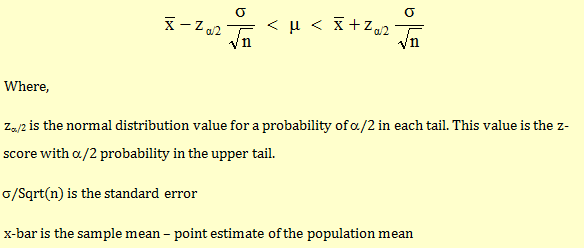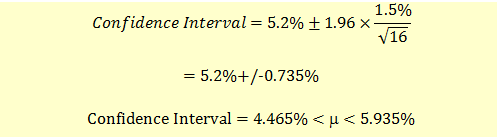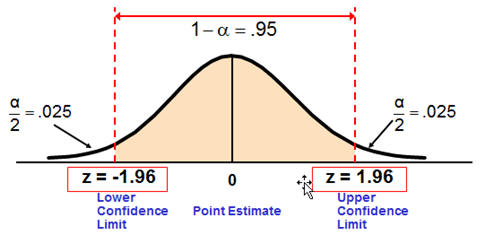# Confidence Interval for a Population mean, with a known Population Variance

We have the following assumptions:

• Population variance σ2 is known
• Population is normally distributed

Under these assumptions, the confidence interval estimate will be given as follows:Example

Let’s take an example to compute this.

We take a sample of 16 stocks from a large population with a mean return of 5.2%. We know that the population standard deviation is 1.5%.

Calculate the 95% confidence interval for the population mean.

For 95% confidence interval, zα/2 = 1.96

The confidence interval will be:We are 95% confidence that the true mean is between 4.465% an­­d 5.935%.

z is obtained from the standard normal distribution table as shown below. F(Z) value is 0.025 at z = -1.96 and F(Z) value is 0.9750 at z = 1.96.The most commonly used confidence intervals are 90%, 95%, 99% and 99.9%. The z values are given below.

 Confidence Level Za/2 Value 90% 1.645 95% 1.96 99% 2.58 99.9% 3.27

Get our Data Science for Finance Bundle for just $29$51.